Successfully reported this slideshow.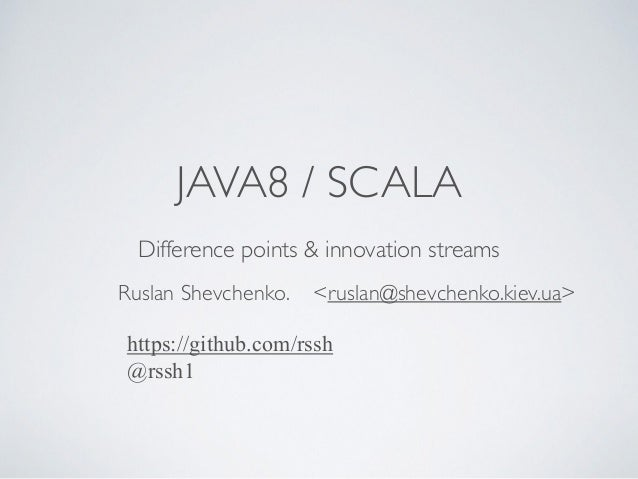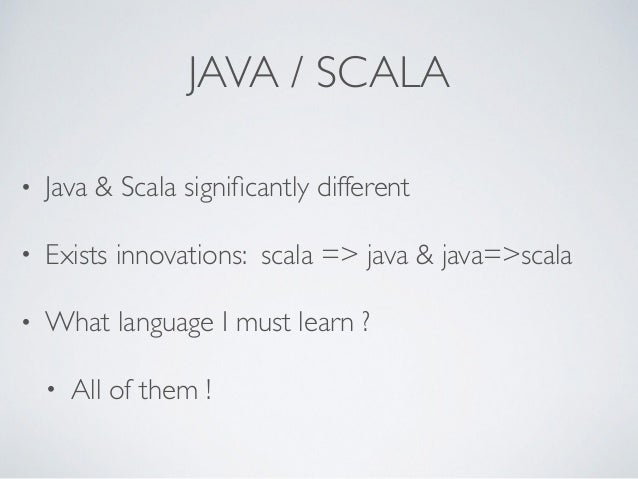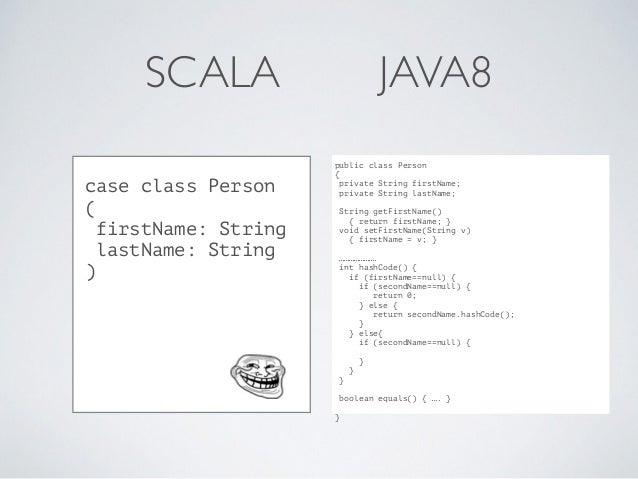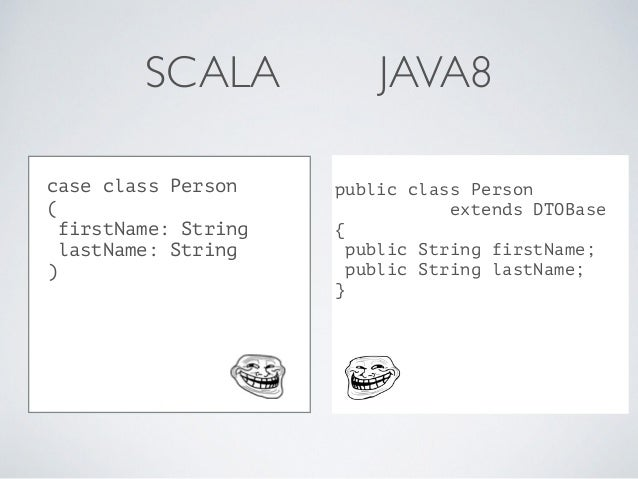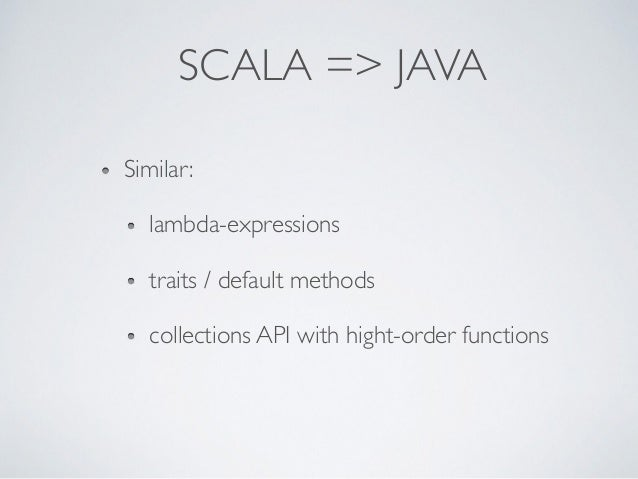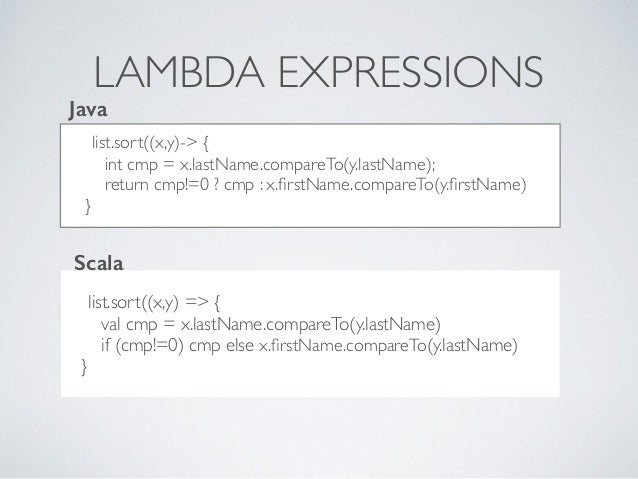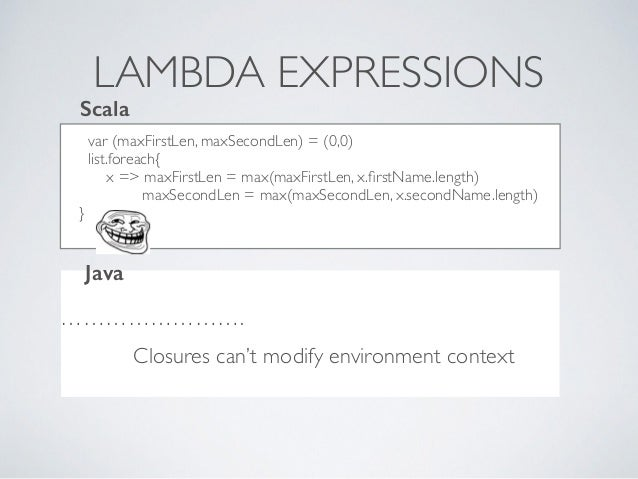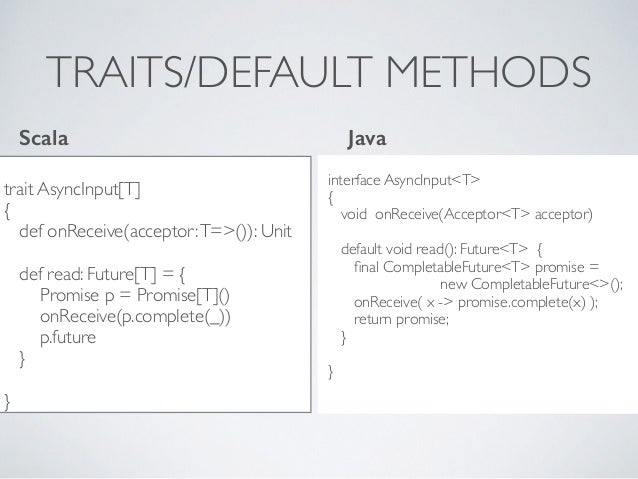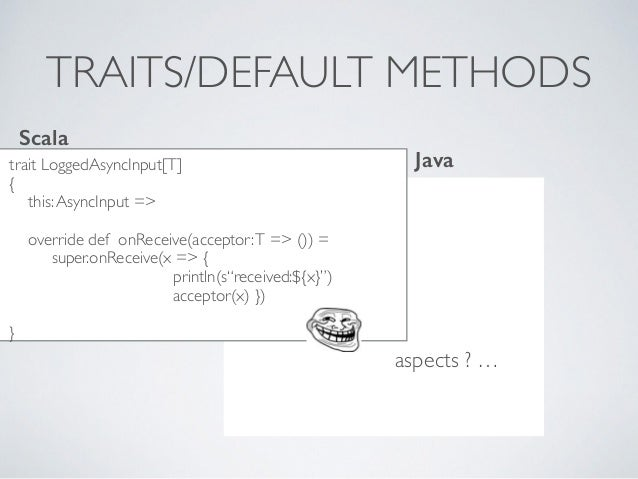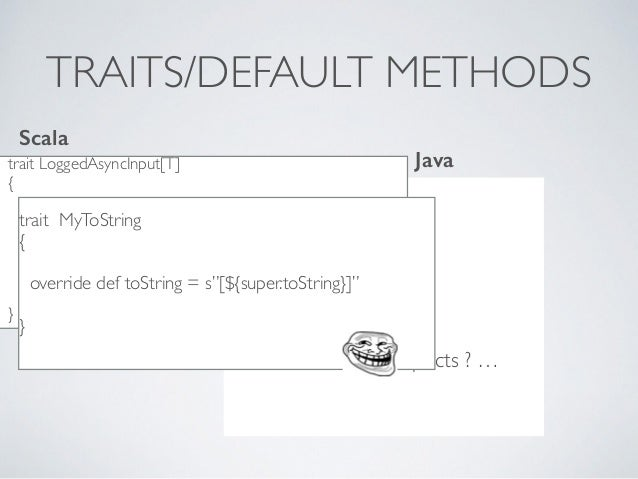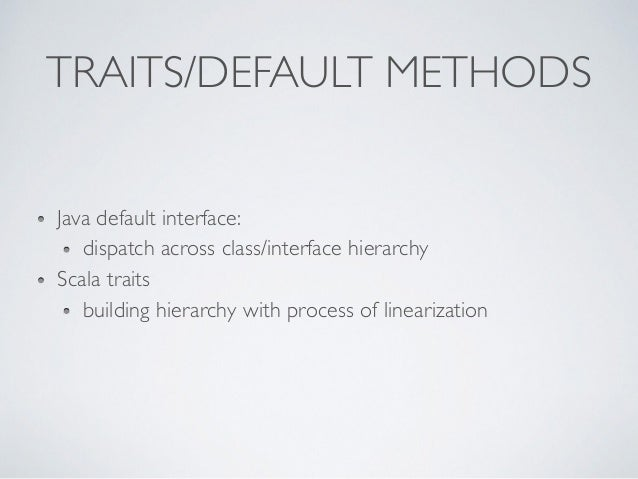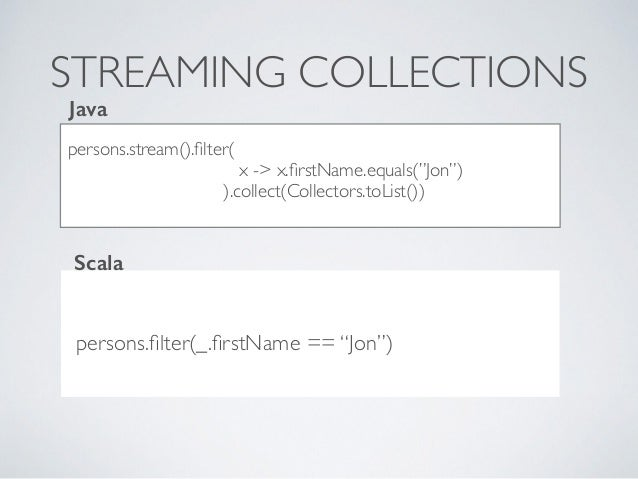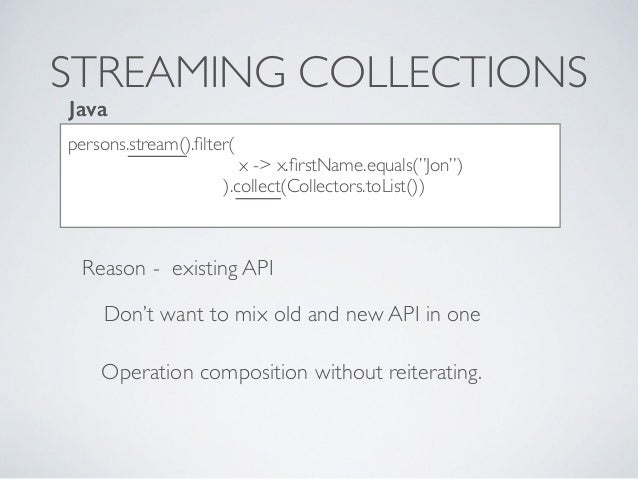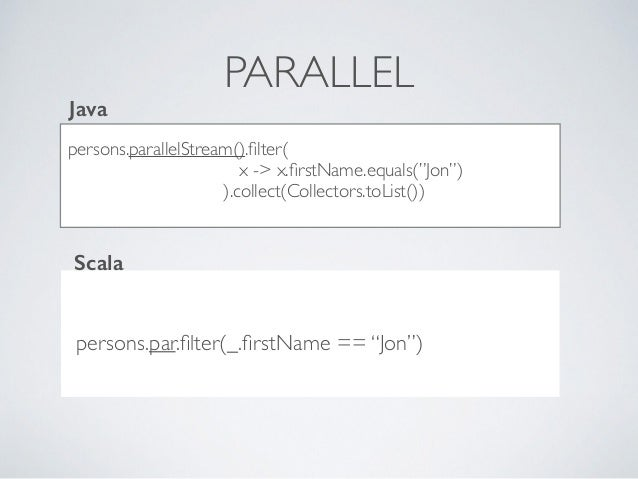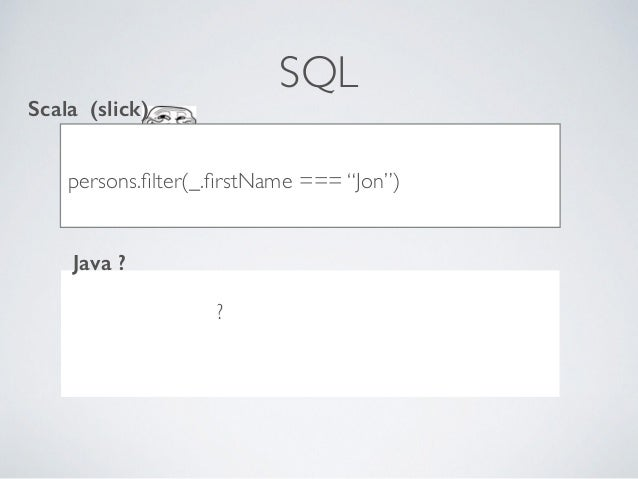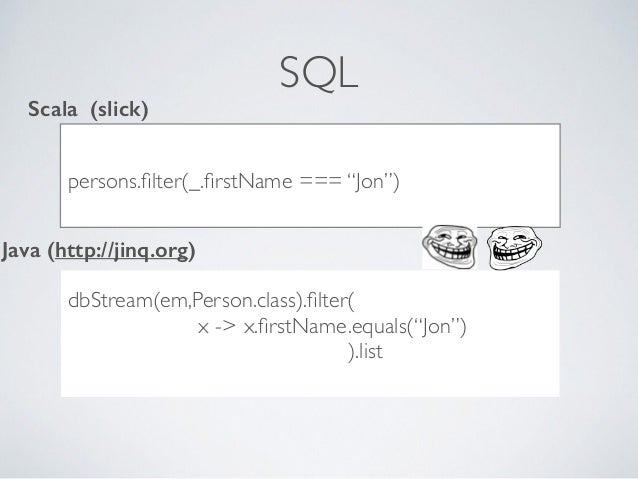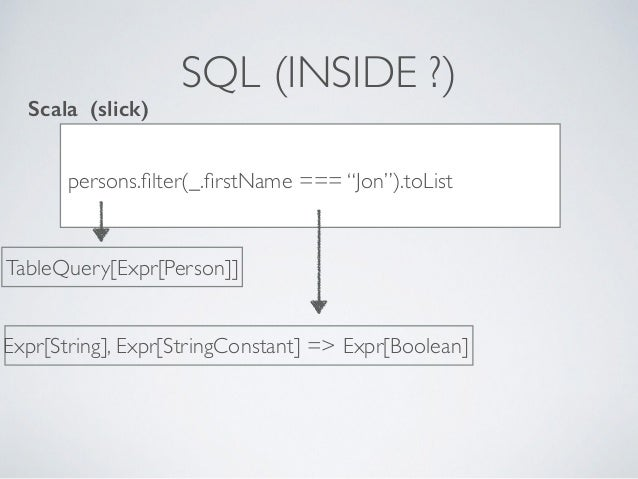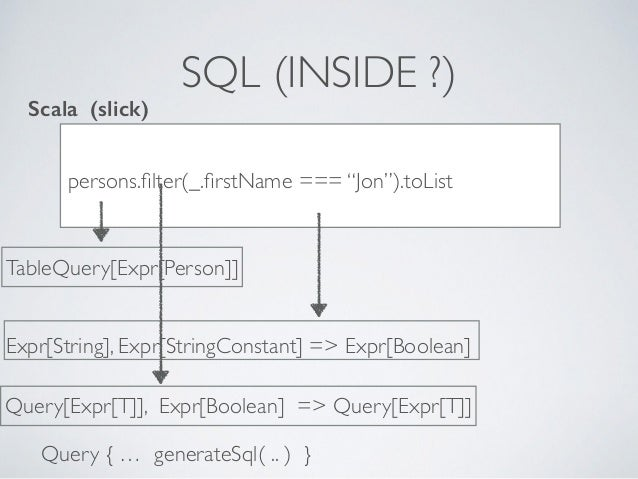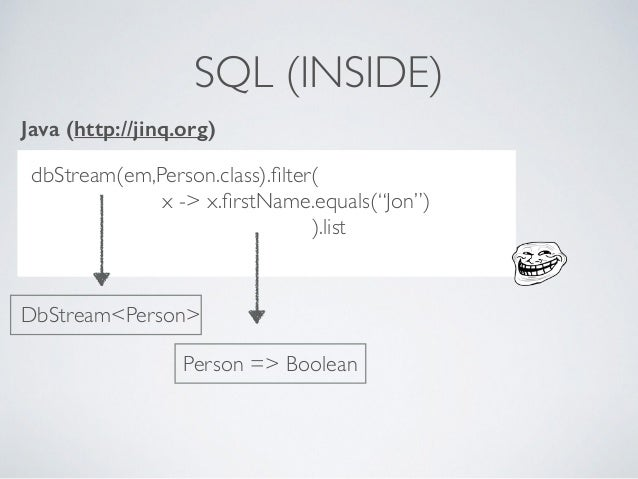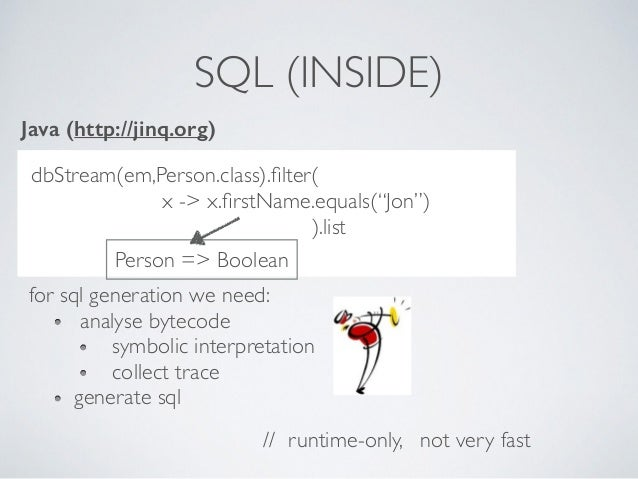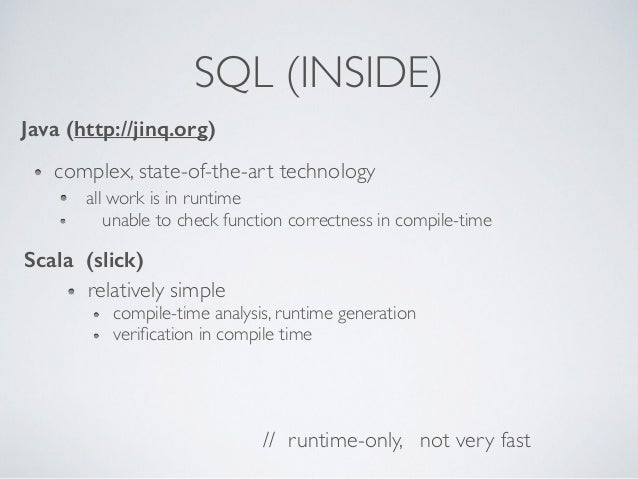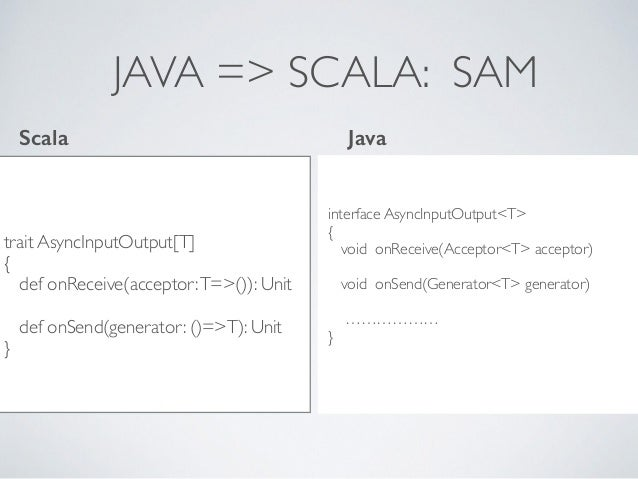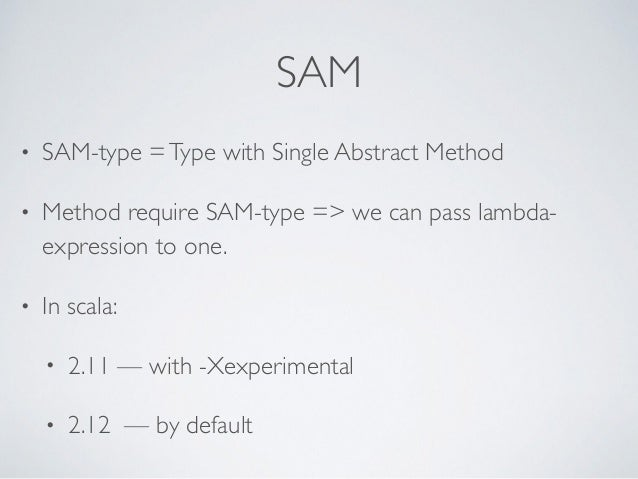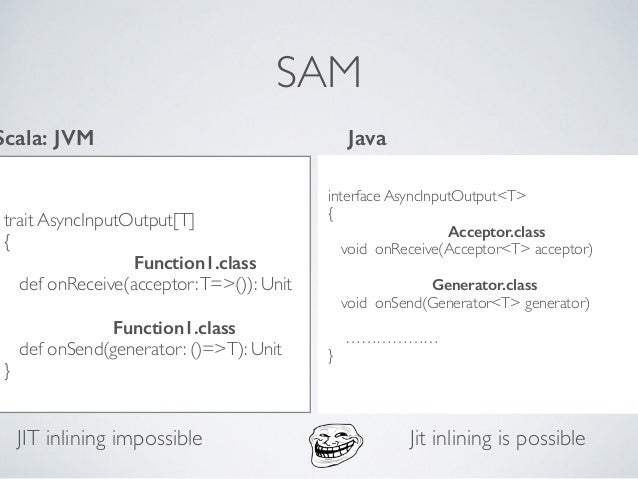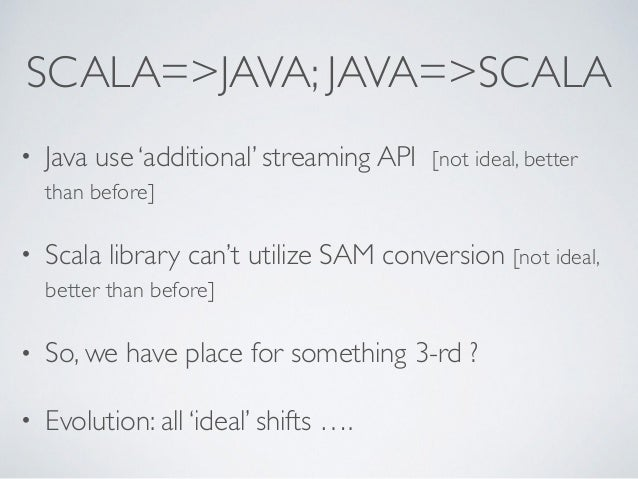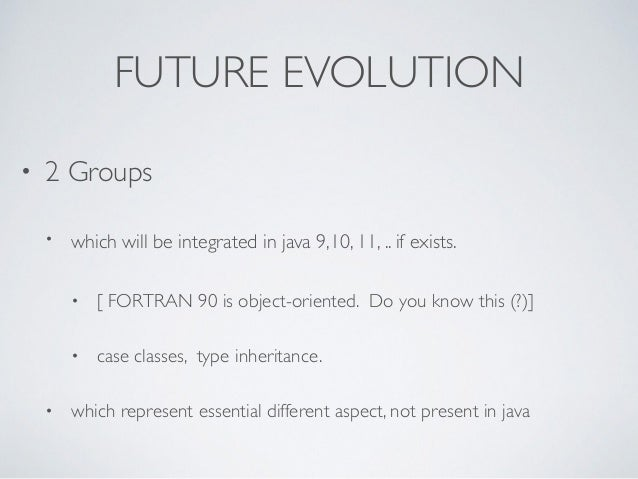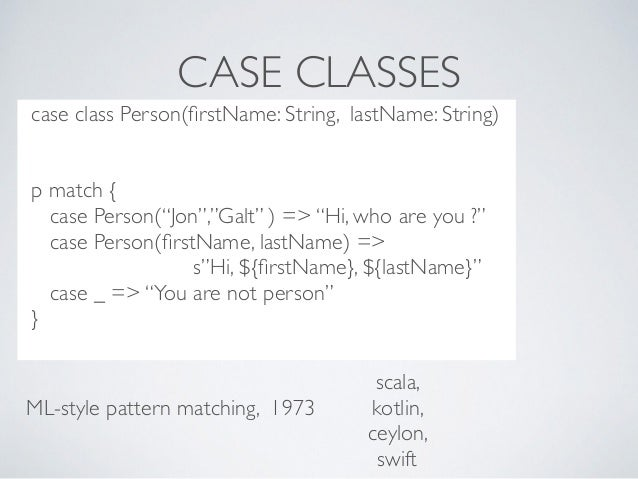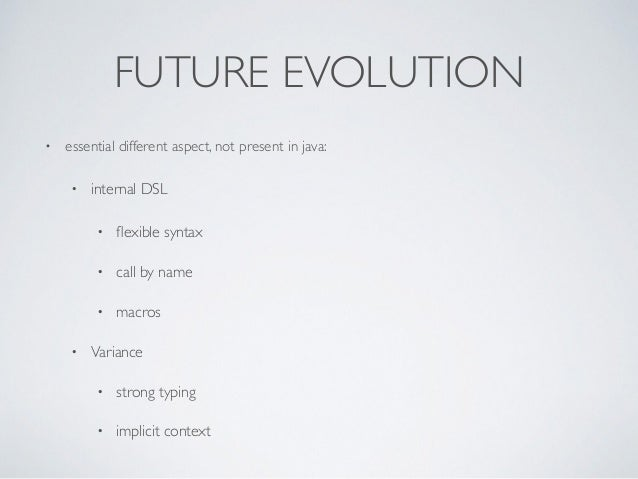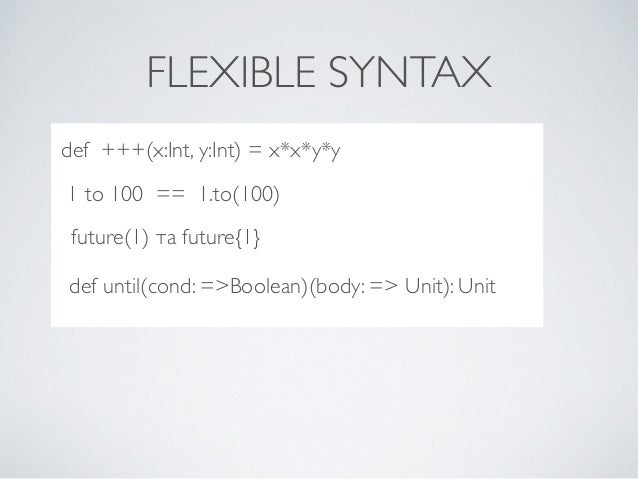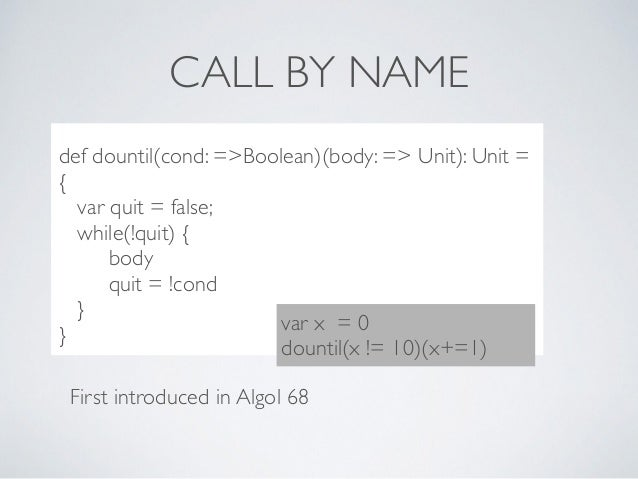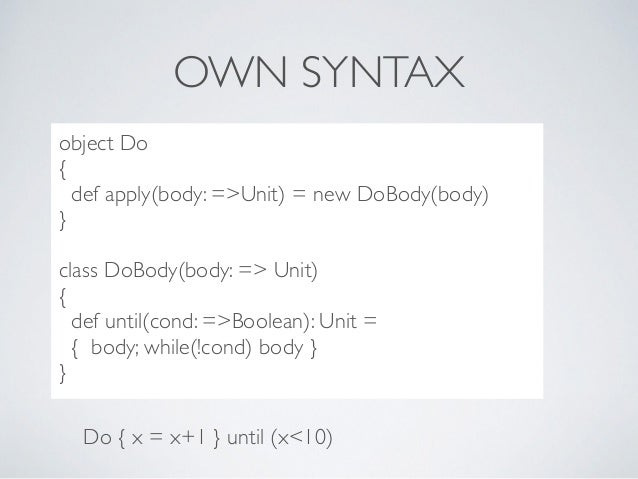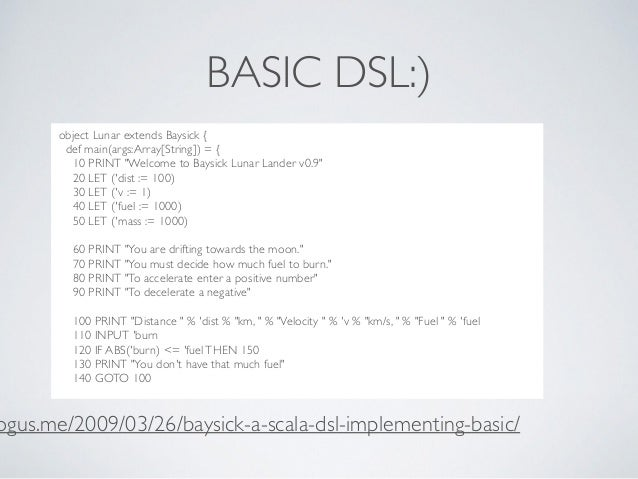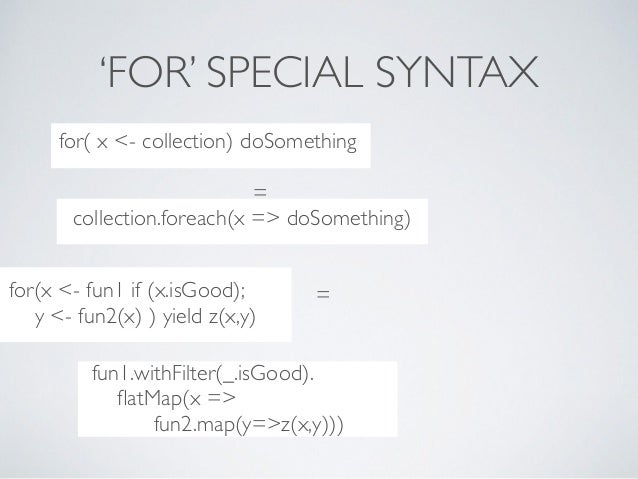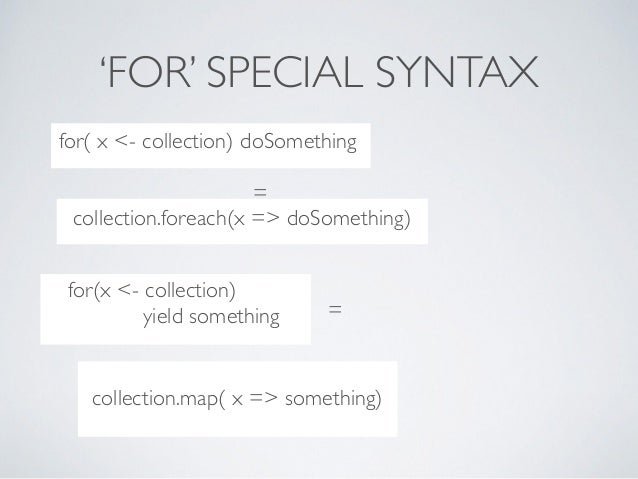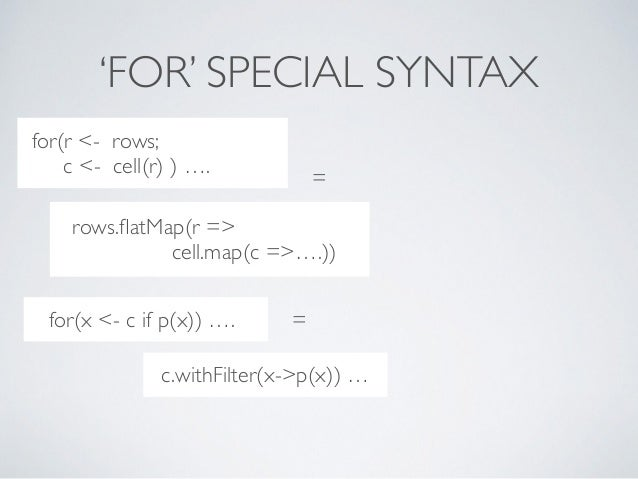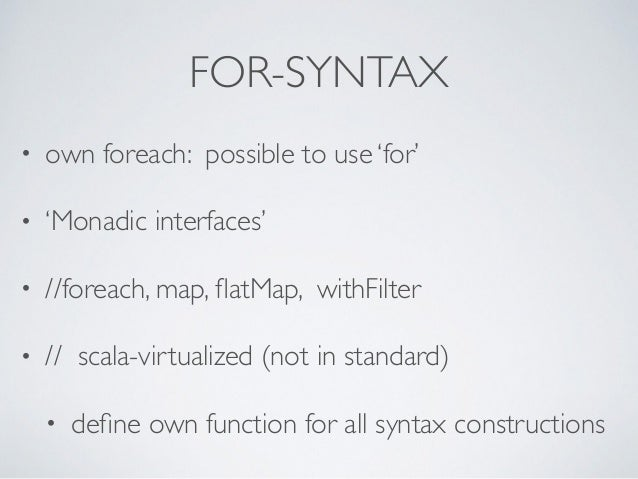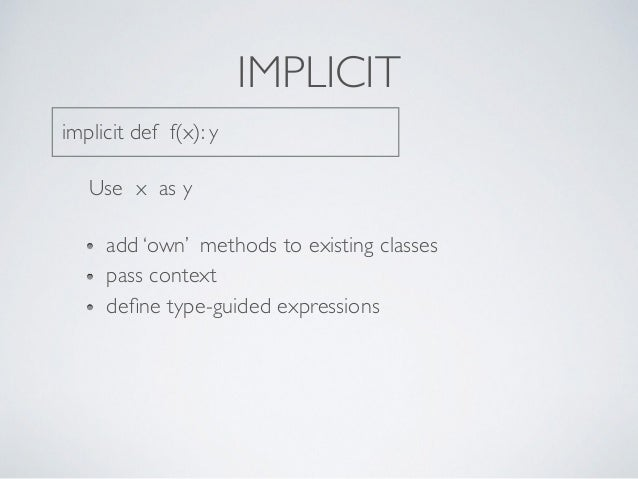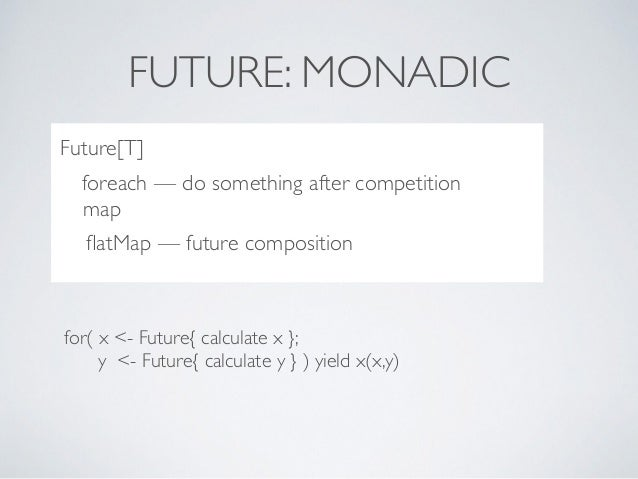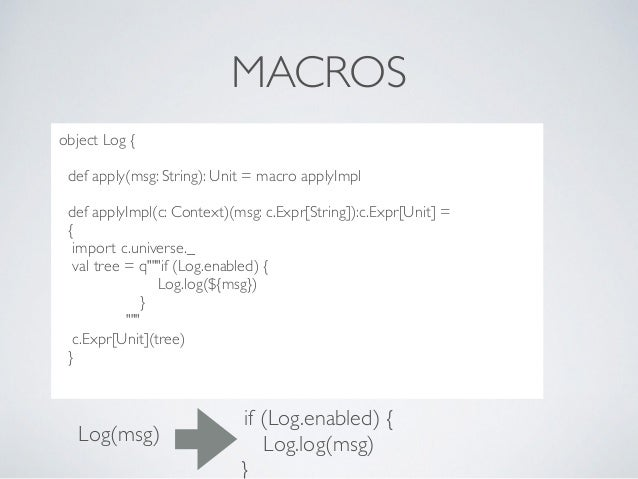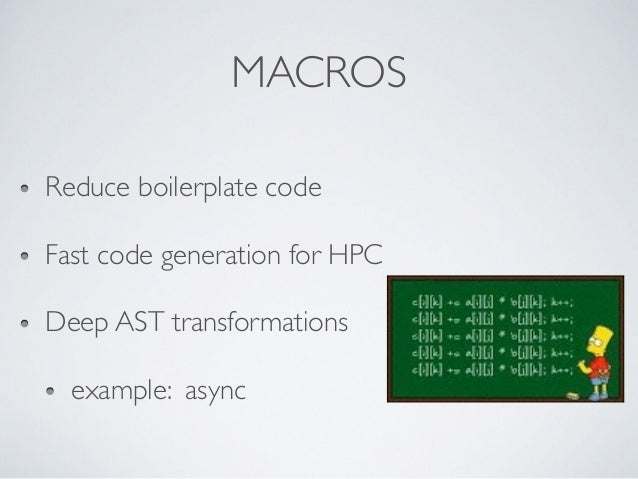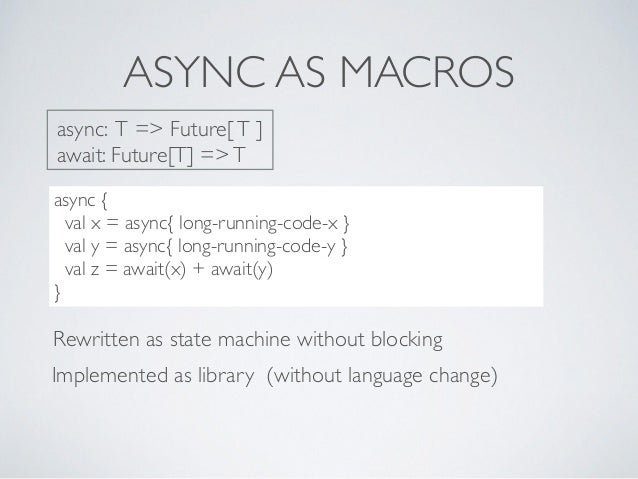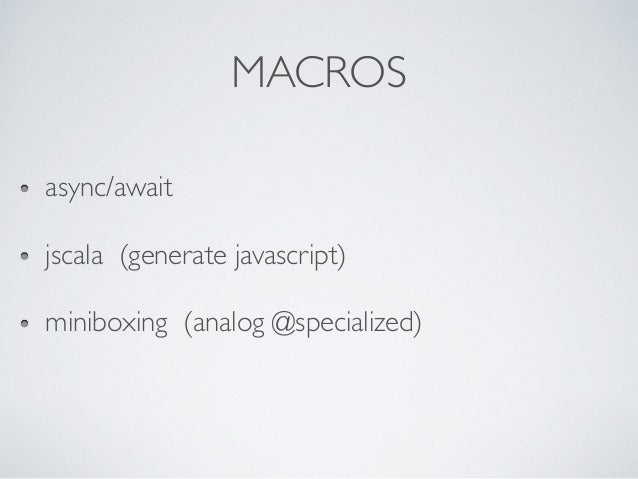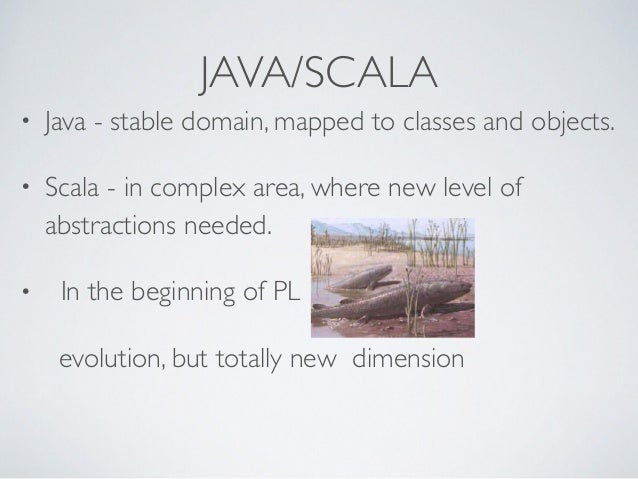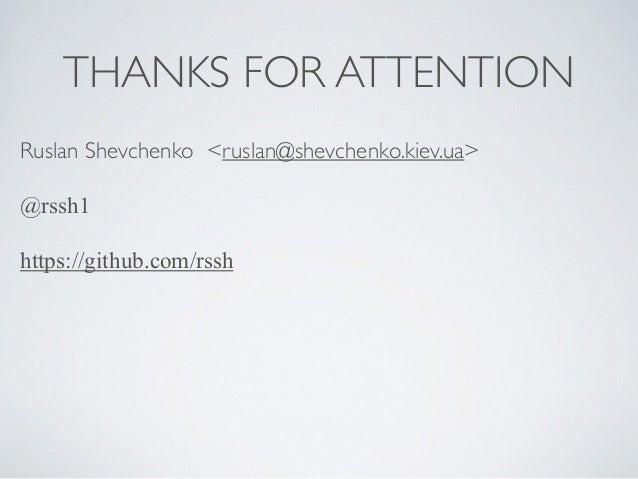Upcoming SlideShare
×
• Full Name
Comment goes here.

Are you sure you want to Yes No• Be the first to comment

### JDays Lviv 2014: Java8 vs Scala: Difference points & innovation stream

1. 1. JAVA8 / SCALA Difference points & innovation streams Ruslan Shevchenko. <ruslan@shevchenko.kiev.ua> https://github.com/rssh @rssh1
2. 2. JAVA / SCALA • Java & Scala signiﬁcantly different • Exists innovations: scala => java & java=>scala • What language I must learn ? • All of them !
3. 3. SCALA JAVA8 public class Person { private String firstName; private String lastName; String getFirstName() { return firstName; } void setFirstName(String v) { firstName = v; } …………………. int hashCode() { if (firstName==null) { if (secondName==null) { return 0; } else { return secondName.hashCode(); } } else{ if (secondName==null) { } } } boolean equals() { …. } } case class Person ( firstName: String lastName: String )
4. 4. SCALA JAVA8 public class Person extends DTOBase { public String firstName; public String lastName; } case class Person ( firstName: String lastName: String )
5. 5. SCALA => JAVA Similar: lambda-expressions traits / default methods collections API with hight-order functions
6. 6. LAMBDA EXPRESSIONS list.sort((x,y)-> { int cmp = x.lastName.compareTo(y.lastName); return cmp!=0 ? cmp : x.ﬁrstName.compareTo(y.ﬁrstName) } list.sort((x,y) => { val cmp = x.lastName.compareTo(y.lastName) if (cmp!=0) cmp else x.ﬁrstName.compareTo(y.lastName) } Java Scala
7. 7. LAMBDA EXPRESSIONS var (maxFirstLen, maxSecondLen) = (0,0) list.foreach{ x => maxFirstLen = max(maxFirstLen, x.ﬁrstName.length) maxSecondLen = max(maxSecondLen, x.secondName.length) } Java Scala Closures can’t modify environment context …………………….
8. 8. TRAITS/DEFAULT METHODS trait AsyncInput[T] { def onReceive(acceptor:T=>()): Unit def read: Future[T] = { Promise p = Promise[T]() onReceive(p.complete(_)) p.future } } interface AsyncInput<T> { void onReceive(Acceptor<T> acceptor) default void read(): Future<T> { ﬁnal CompletableFuture<T> promise = new CompletableFuture<>(); onReceive( x -> promise.complete(x) ); return promise; } } Scala Java
9. 9. TRAITS/DEFAULT METHODS trait LoggedAsyncInput[T] { this:AsyncInput => override def onReceive(acceptor:T => ()) = super.onReceive(x => { println(s“received:\${x}”) acceptor(x) }) } Scala Java aspects ? …
10. 10. TRAITS/DEFAULT METHODS trait LoggedAsyncInput[T] { override def onReceive(acceptor:T => ()) = super.onReceive(x => { println(s“received:\${x}”) acceptor(x) }) } Scala Java aspects ? … trait MyToString { override def toString = s”[\${super.toString}]” }
11. 11. TRAITS/DEFAULT METHODS Java default interface: dispatch across class/interface hierarchy Scala traits building hierarchy with process of linearization
12. 12. STREAMING COLLECTIONS persons.stream().ﬁlter( x -> x.ﬁrstName.equals(”Jon”) ).collect(Collectors.toList()) persons.ﬁlter(_.ﬁrstName == “Jon”) Java Scala
13. 13. STREAMING COLLECTIONS persons.stream().ﬁlter( x -> x.ﬁrstName.equals(”Jon”) ).collect(Collectors.toList()) Java Reason - existing API Don’t want to mix old and new API in one Operation composition without reiterating.
14. 14. PARALLEL persons.parallelStream().ﬁlter( x -> x.ﬁrstName.equals(”Jon”) ).collect(Collectors.toList()) persons.par.ﬁlter(_.ﬁrstName == “Jon”) Java Scala
15. 15. SQL ..??***8dc persons.ﬁlter(_.ﬁrstName === “Jon”) Scala (slick) Java ? ?
16. 16. SQL ..??***8dc persons.ﬁlter(_.ﬁrstName === “Jon”) Scala (slick) Java (http://jinq.org) dbStream(em,Person.class).ﬁlter( x -> x.ﬁrstName.equals(“Jon”) ).list
17. 17. SQL (INSIDE ?) persons.ﬁlter(_.ﬁrstName === “Jon”).toList Scala (slick) TableQuery[Expr[Person]] Expr[String], Expr[StringConstant] => Expr[Boolean]
18. 18. SQL (INSIDE ?) persons.ﬁlter(_.ﬁrstName === “Jon”).toList Scala (slick) TableQuery[Expr[Person]] Expr[String], Expr[StringConstant] => Expr[Boolean] Query[Expr[T]], Expr[Boolean] => Query[Expr[T]] Query { … generateSql( .. ) }
19. 19. SQL (INSIDE) ..??***8dc Java (http://jinq.org) dbStream(em,Person.class).ﬁlter( x -> x.ﬁrstName.equals(“Jon”) ).list DbStream<Person> Person => Boolean
20. 20. SQL (INSIDE) ..??***8dc Java (http://jinq.org) dbStream(em,Person.class).ﬁlter( x -> x.ﬁrstName.equals(“Jon”) ).list Person => Boolean for sql generation we need: analyse bytecode symbolic interpretation collect trace generate sql // runtime-only, not very fast
21. 21. SQL (INSIDE) Java (http://jinq.org) // runtime-only, not very fast complex, state-of-the-art technology all work is in runtime unable to check function correctness in compile-time Scala (slick) relatively simple compile-time analysis, runtime generation veriﬁcation in compile time
22. 22. JAVA => SCALA: SAM trait AsyncInputOutput[T] { def onReceive(acceptor:T=>()): Unit def onSend(generator: ()=>T): Unit } interface AsyncInputOutput<T> { void onReceive(Acceptor<T> acceptor) void onSend(Generator<T> generator) ……………… } Scala Java
23. 23. SAM • SAM-type =Type with Single Abstract Method • Method require SAM-type => we can pass lambda- expression to one. • In scala: • 2.11 — with -Xexperimental • 2.12 — by default
24. 24. SAM trait AsyncInputOutput[T] { Function1.class def onReceive(acceptor:T=>()): Unit Function1.class def onSend(generator: ()=>T): Unit } interface AsyncInputOutput<T> { Acceptor.class void onReceive(Acceptor<T> acceptor) Generator.class void onSend(Generator<T> generator) ……………… } Scala: JVM Java JIT inlining impossible Jit inlining is possible
25. 25. SCALA=>JAVA; JAVA=>SCALA • Java use ‘additional’ streaming API [not ideal, better than before] • Scala library can’t utilize SAM conversion [not ideal, better than before] • So, we have place for something 3-rd ? • Evolution: all ‘ideal’ shifts ….
26. 26. FUTURE EVOLUTION • 2 Groups • which will be integrated in java 9,10, 11, .. if exists. • [ FORTRAN 90 is object-oriented. Do you know this (?)] • case classes, type inheritance. • which represent essential different aspect, not present in java
27. 27. CASE CLASSES ..??***8dc case class Person(ﬁrstName: String, lastName: String) p match { case Person(“Jon”,”Galt” ) => “Hi, who are you ?” case Person(ﬁrstName, lastName) => s”Hi, \${ﬁrstName}, \${lastName}” case _ => “You are not person” } ML-style pattern matching, 1973 scala, kotlin, ceylon, swift
28. 28. FUTURE EVOLUTION • essential different aspect, not present in java: • internal DSL • ﬂexible syntax • call by name • macros • Variance • strong typing • implicit context
29. 29. FLEXIBLE SYNTAX ..??***8dc def +++(x:Int, y:Int) = x*x*y*y 1 to 100 == 1.to(100) future(1) та future{1} def until(cond: =>Boolean)(body: => Unit): Unit
30. 30. CALL BY NAME ..??***8dc def dountil(cond: =>Boolean)(body: => Unit): Unit = { var quit = false; while(!quit) { body quit = !cond } } First introduced in Algol 68 var x = 0 dountil(x != 10)(x+=1)
31. 31. OWN SYNTAX ..??***8dc object Do { def apply(body: =>Unit) = new DoBody(body) } class DoBody(body: => Unit) { def until(cond: =>Boolean): Unit = { body; while(!cond) body } } Do { x = x+1 } until (x<10)
32. 32. BASIC DSL:) ..??***8dc object Lunar extends Baysick { def main(args:Array[String]) = { 10 PRINT "Welcome to Baysick Lunar Lander v0.9" 20 LET ('dist := 100) 30 LET ('v := 1) 40 LET ('fuel := 1000) 50 LET ('mass := 1000) 60 PRINT "You are drifting towards the moon." 70 PRINT "You must decide how much fuel to burn." 80 PRINT "To accelerate enter a positive number" 90 PRINT "To decelerate a negative" 100 PRINT "Distance " % 'dist % "km, " % "Velocity " % 'v % "km/s, " % "Fuel " % 'fuel 110 INPUT 'burn 120 IF ABS('burn) <= 'fuelTHEN 150 130 PRINT "You don't have that much fuel" 140 GOTO 100 ogus.me/2009/03/26/baysick-a-scala-dsl-implementing-basic/
33. 33. ..??***8dccollection.foreach(x => doSomething) ‘FOR’ SPECIAL SYNTAX ..??***8dcfor( x <- collection) doSomething = ..??***8dc for(x <- fun1 if (x.isGood); y <- fun2(x) ) yield z(x,y) = ..??***8dc fun1.withFilter(_.isGood). ﬂatMap(x => fun2.map(y=>z(x,y)))
34. 34. ..??***8dccollection.foreach(x => doSomething) ‘FOR’ SPECIAL SYNTAX ..??***8dcfor( x <- collection) doSomething = ..??***8dc for(x <- collection) yield something = ..??***8dccollection.map( x => something)
35. 35. ‘FOR’ SPECIAL SYNTAX ..??***8dc = ..??**8dc for(r <- rows; c <- cell(r) ) …. rows.ﬂatMap(r => cell.map(c =>….)) ..??***8dcfor(x <- c if p(x)) …. = ..??***8dcc.withFilter(x->p(x)) …
36. 36. ..??***8dccollection.foreach(x => doSomething) ‘FOR’ SPECIAL SYNTAX ..??***8dcfor( x <- collection) doSomething = ..??***8dc for(x <- fun1 if (x.isGood); y <- fun2(x) ) yield z(x,y) = ..??***8dc fun1.withFilter(_.isGood). ﬂatMap(x => fun2.map(y=>z(x,y)))
37. 37. FOR-SYNTAX • own foreach: possible to use ‘for’ • ‘Monadic interfaces’ • //foreach, map, ﬂatMap, withFilter • // scala-virtualized (not in standard) • deﬁne own function for all syntax constructions
38. 38. IMPLICIT implicit def f(x): y Use x as y add ‘own’ methods to existing classes pass context deﬁne type-guided expressions
39. 39. FUTURE: MONADIC ..??***8dc for( x <- Future{ calculate x }; y <- Future{ calculate y } ) yield x(x,y) Future[T] foreach — do something after competition map ﬂatMap — future composition
40. 40. MACROS ..??***8dc object Log { def apply(msg: String): Unit = macro applyImpl def applyImpl(c: Context)(msg: c.Expr[String]):c.Expr[Unit] = { import c.universe._ val tree = q"""if (Log.enabled) { Log.log(\${msg}) } """ c.Expr[Unit](tree) } Log(msg) if (Log.enabled) { Log.log(msg) }
41. 41. MACROS Reduce boilerplate code Fast code generation for HPC Deep AST transformations example: async
42. 42. ASYNC AS MACROS ..??***8dc async { val x = async{ long-running-code-x } val y = async{ long-running-code-y } val z = await(x) + await(y) } Rewritten as state machine without blocking Implemented as library (without language change) async: T => Future[T ] await: Future[T] =>T
43. 43. MACROS async/await jscala (generate javascript) miniboxing (analog @specialized)
44. 44. JAVA/SCALA • Java - stable domain, mapped to classes and objects. • Scala - in complex area, where new level of abstractions needed. • In the beginning of PL evolution, but totally new dimension